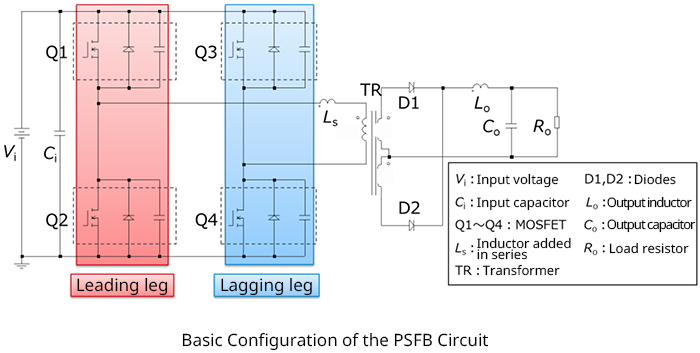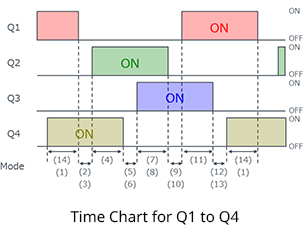# Basic Configuration of a PSFB Circuit

2021.09.15

・Phase shift full bridge (PSFB) circuits make possible dramatic reductions in switching losses through zero-voltage switching (ZVS) operation of switching elements, and so can handle greater amounts of power.

・A PSFB switching circuit essentially consists of four switching elements (MOSFETs); the leakage inductance of a transformer is used as a resonance inductance necessary for ZVS operation.

・In order to extend the range of ZVS operation, there are cases in which an inductor is added in series. Here, a circuit with an added series inductor is assumed.

・In the basic switching operation, after the on/off states of Q1 and Q2 have been swapped, the on/off states of Q3 and Q4 are swapped after a certain phase lag.

・In general, the leg of Q1 and Q2 is called the "leading leg", and the leg of Q3 and Q4 is the "lagging leg".

## Basic Configuration of a PSFB Circuit

To begin with, the basic configuration of a PSFB circuit is presented. Upon grasping this basic configuration, the circuit operation, described below, should also be easy to understand.The leakage inductance of the transformer may be utilized as a resonance inductor in order to realize ZVS, which is one feature of the PSFB configuration; however, in some cases an inductor is added in series with the transformer to broaden the range of ZVS operation. Here, a circuit is assumed in which an added series inductor LS is used.

Next, the on/off timing chart for the switches is shown. The figures appearing in the bottom below the bidirectional arrows are labels representing the circuit operation modes. These correspond to the mode numbers used in the following explanation of circuit operation.

As shown in the chart, after the on/off states of Q1 and Q2 have been swapped, the on/off states of Q3 and Q4 are swapped with a certain phase lag. From this fact, the leg of Q1 and Q2 is generally called the “leading leg”, and the leg of Q3 and Q4 is the “lagging leg”.